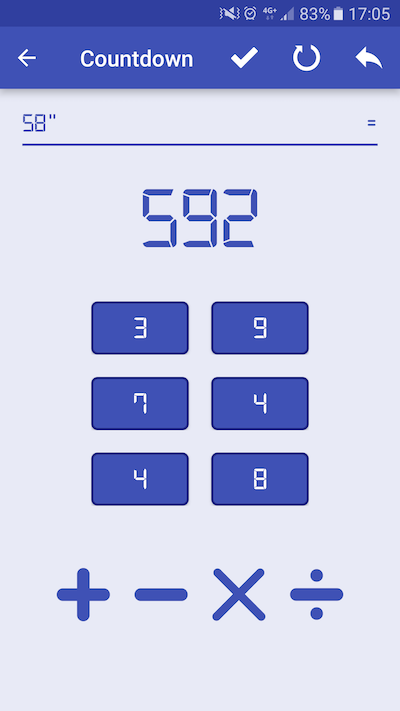The Countdown Math Game, also known as Good Count Math Game, is a game made famous in England with the TV game show Countdown which started in the sixties and then exported in a lot of countries around the World.The Countdown Math Game involves number puzzles in which you must make arithmetic operations to reach a random target number (between 100 and 999) from 6 other numbers chosen randomly (from 1 to 10, 25, 50, 75 and 100). In this tutorial, you are going to create your own implementation of a solver for the Countdown Math Game on Android.

Note that you can discover this tutorial in video on YouTube :

You can also test directly an extended version of the Countdown Math Game we are going to create in this tutorial just here on the Google Play Store :

## Modelize the Countdown Math Game

Before starting to create the algorithm to solve the Countdown Math Game, we are going to modelize the game. Like said previously, you can use the four primary artihmetic operations to solve a count :

• Addition
• Subtraction
• Division
• Multiplication

Basically, each operation take two parameters and return a result. Besides, for each operation we have an associated symbol. So, we can create an Operation interface to modelize the behaviour of an operation in the Countdown Math Game :

Then, we need to implement each operation in a dedicated class implementing the Operation interface :

• Add
• Subtract
• Divide
• Multiply

## Algorithm to solve the Countdown Math Game

Now, we can create an algorithm to solve the Countdown Math Game. We are going to create a brute force algorithm iterating on all the numbers to use and applying all the possible operation to reach the target number.

Our algorithm will have the following form :

You can note that we are going to use a list to store all the operations leading to a solution of a count to find. The printSolution method will just have to iterate on the list in the reverse way to display the operations leading to the number to reach.

## Create the User Interface

With our CountdownMathGame object implemented, we have to create the User Interface of our game on Android. The UI will be quite simple with mainly two EditText components letting the users respectively to enter the number to use separated by a space and the number to reach. The last view will be a Button which will be useful to start the resolution of the Countdown Math Game.

Our User Interface will have the following form :

You can see a preview of the User Interface just below :## Write the code of the Main Activity

Now, we can write the Java code of the Main Activity of our Countdown Math Game for Android. We just need to get the references of the UI’s views and then to set an OnClickListener implementation on the Button. When the user will click on it, we will have to get the numbers to use and to parse it from String to Integer. Then, we will get the number to reach from the second EditText.

With these numbers, we could launch the resolution of the Countdown Math Game by calling the findSolution method. We will put the numbers to use and the number to reach in parameter of this method.

This gives us the following code for the Main Activity :

Here, you can note that if there is a solution, we show it to the user in an AlertDialog. It is the main difference with the CountdownMathGame class presented previously in which we print the solution on standard output.Our Countdown Math Game Solver for Android works great ! You can discover the algorithm implemented in this tutorial in action just here on YouTube :

That’s all for that tutorial. To discover more tutorials on Android development, don’t hesitate to visit the SSaurel’s Channel on YouTube :  https://www.youtube.com/user/sylsau/You can also visit the SSaurel’s Blog : https://www.ssaurel.com/blog